Lines and Angles
Angle Relationships
Find That Angle
Triangle Theorems
Equations of Lines
100

Name a line segment parallel to line RVTX, SW, or QU

100

The angles #1 and #5 are ____?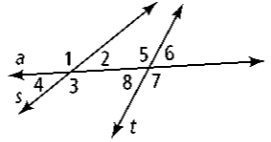Corresponding angles

100

What is the angle measure of #7 and the theorem to justify it?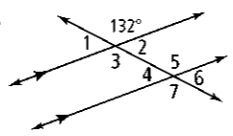132 degrees and Alternate Exterior Angles Theorem

100

What is the measure of angle 1?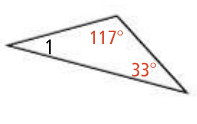30 degrees

100

Find the slope of the line graphed below.- 5/6

200

Name any pair of parallel planes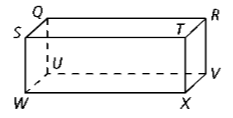TRVX and SQUW, STXW and QRVU, or SQRT and WUVX

200

Angles #3 and #8 are ____?Same-side interior angles

200

What is the angle measure of #2 and the theorem to justify it?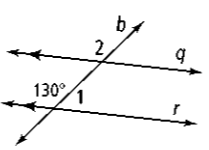130 degrees and Corresponding Angles Theorem

200

What is the measure of angle 1?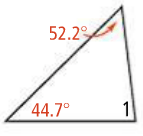83.1 degrees

200

Find the slope of the line through the points

(-6, 2) and (-7, 10).

-8

300

Name all lines that are parallel to line WXST,  QR,  and  UV

300

Angles #4 and #6 are ____?Alternate exterior angles

300

What is the angle measure of #2 and the theorem to justify it?48 degrees and the Linear Pair Theorem

300

What is the measure of angle 1?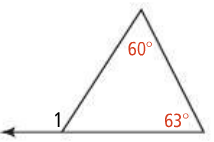123 degrees

300

What is the point-slope form of an equation?

y - y1 = m(x - x1)

400

Name four lines that are skew (different plane) to RV.SQ,  WU,  WX, and ST

400

Name all pairs of alternate exterior angles.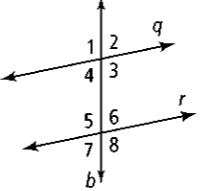1 & 8 and 2 & 7

400

What is the angle measure of #1 and the theorem to justify it?50 degrees and the Linear Pair Theorem

400

What is the measure of angle a?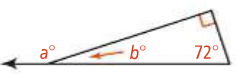162 degrees

400

What is the equation of a line with slope 3 and y-intercept 6?

y = 3x + 6

500

Describe a real world example of either parallel or skew lines or planes.

Ex: Two roads going parallel. The top left edge of a building and the right side of a building.

500

Name all pairs of corresponding angles.1 & 5, 2 & 6, 3 & 8, and 4 & 7

500

What is the measure of angle #1 and the theorem to justify it.  And what is the measure of angle #2 and the theorem to justify it.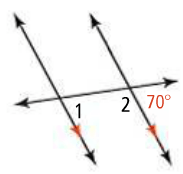70 degrees for #1 using Corresponding Angles Theorem and 110 degrees for #2 using Same-Side Interior Angles Postulate

500

What is the measure of angle y?110 degrees

500

Find the equation of the graphed line below.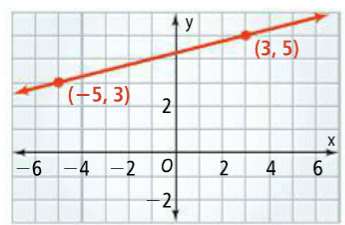y = 1/4(x) + 17/4

or

y = 1/4(x) + 4 1/4

or

y - 3 = 1/4( x + 5 )

or

y - 5 = 1/4 (x - 3)

Click to zoom